# 一遍记住Java常用的八种排序算法与代码实现

2020-12-24 00:27

1. 将第一个数和第二个数排序，然后构成一个有序序列

2. 将第三个数插入进去，构成一个新的有序序列。

3. 对第四个数、第五个数…… 直到最后一个数，重复第二步。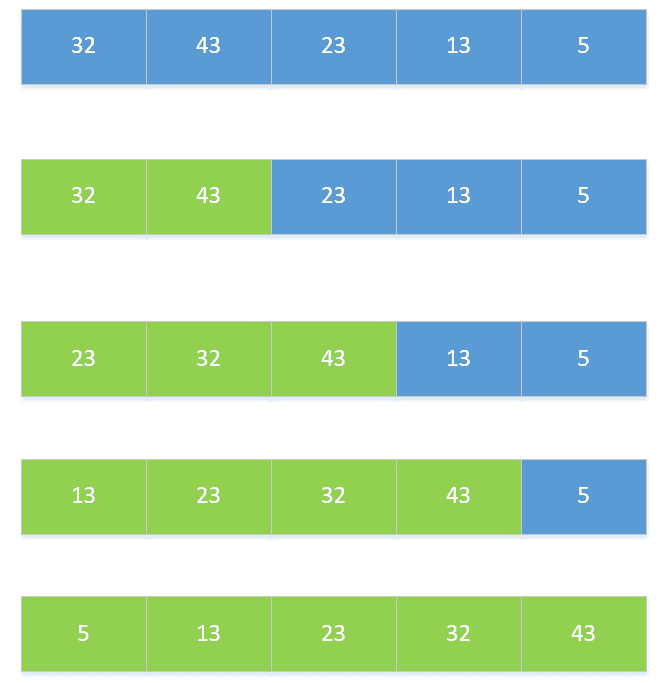1. 首先设定插入次数，即循环次数，for(int i=1;i

2. 设定插入数和得到已经排好序列的最后一个数的位数。insertNum 和 j=i-1。

3. 从最后一个数开始向前循环，如果插入数小于当前数，就将当前数向后移动一位。

4. 将当前数放置到空着的位置，即 j+1。

``````public void insertSort(int[] a){
int length=a.length;
int insertNum;
for(int i=1;i=0&&a[j]>insertNum){
a[j+1]=a[j];
j--;
}
a[j+1]=insertNum;
}
}``````

### 二、希尔排序

1. 将数的个数设为 n，取奇数 k=n/2，将下标差值为 k 的数分为一组，构成有序序列。

2. 再取 k=k/2 ，将下标差值为 k 的书分为一组，构成有序序列。

3. 重复第二步，直到 k=1 执行简单插入排序。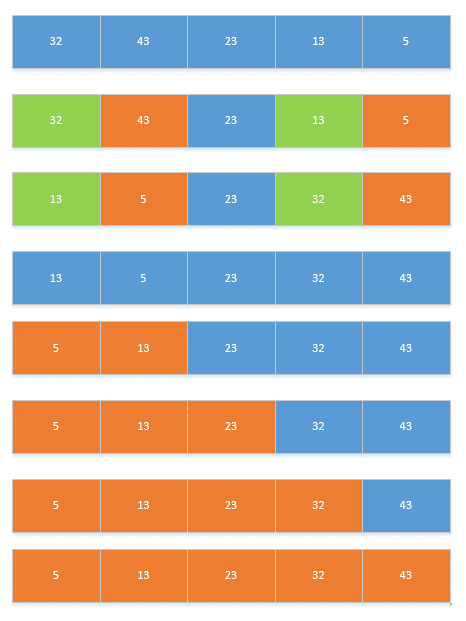1. 首先确定分的组数。

2. 然后对组中元素进行插入排序。

3. 然后将 length/2，重复 1,2 步，直到 length=0 为止。

``````public  void sheelSort(int[] a){
int d  = a.length;
while (d!=0) {
d=d/2;
for (int x = 0; x = 0 && temp ``````

### 三、简单选择排序

(如果每次比较都交换，那么就是交换排序；如果每次比较完一个循环再交换，就是简单选择排序。)

1. 遍历整个序列，将最小的数放在最前面。

2. 遍历剩下的序列，将最小的数放在最前面。

3. 重复第二步，直到只剩下一个数。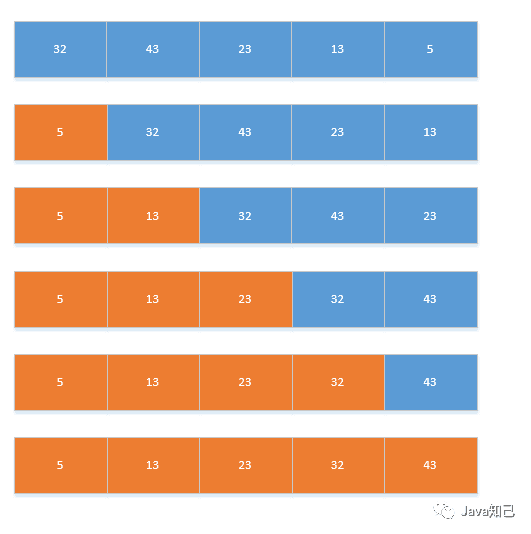1. 首先确定循环次数，并且记住当前数字和当前位置。

2. 将当前位置后面所有的数与当前数字进行对比，小数赋值给 key，并记住小数的位置。

3. 比对完成后，将最小的值与第一个数的值交换。

4. 重复 2、3 步。

``````    public void selectSort(int[] a) {
int length = a.length;
for (int i = 0; i ``````

### 四、堆排序

1. 将序列构建成大顶堆。

2. 将根节点与最后一个节点交换，然后断开最后一个节点。

3. 重复第一、二步，直到所有节点断开。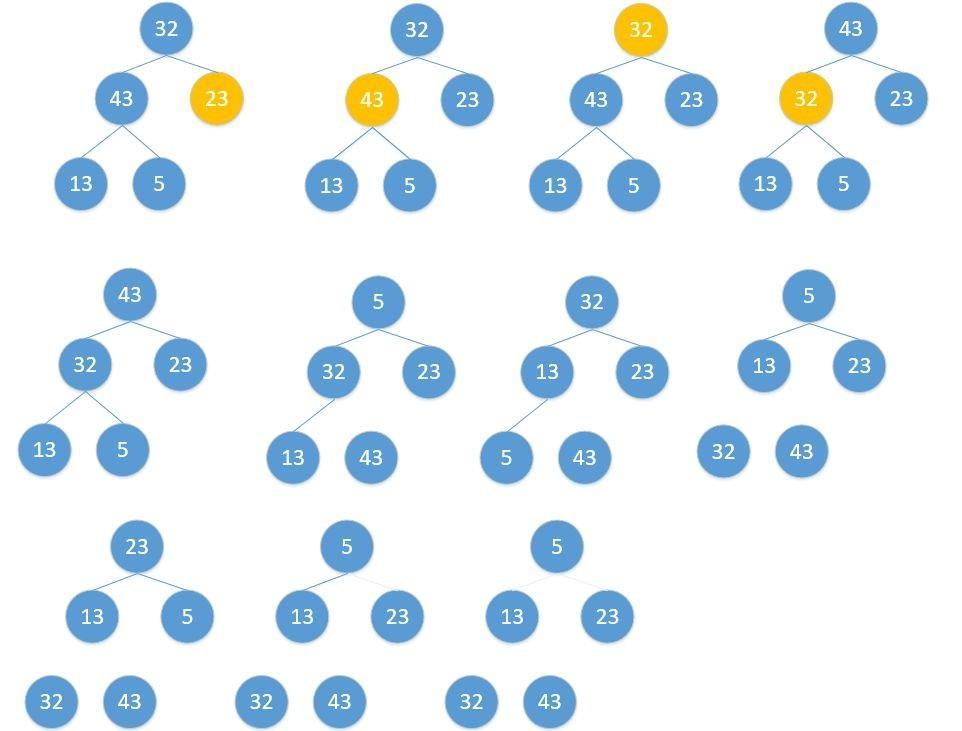``````public  void heapSort(int[] a){
System.out.println("开始排序");
int arrayLength=a.length;

for(int i=0;i=0;i--){

int k=i;

while(k*2+1``````

### 五、冒泡排序

1. 将序列中所有元素两两比较，将最大的放在最后面。

2. 将剩余序列中所有元素两两比较，将最大的放在最后面。

3. 重复第二步，直到只剩下一个数。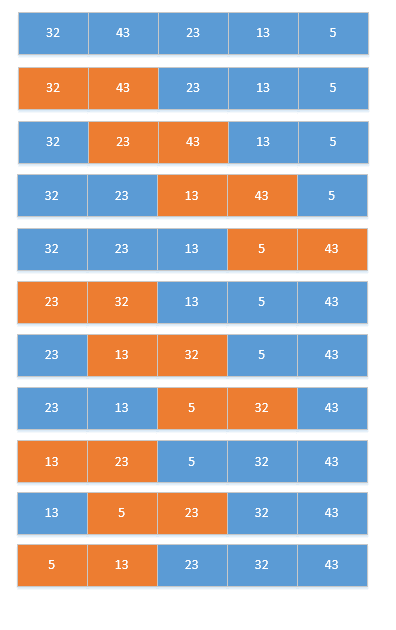1. 设置循环次数。

2. 设置开始比较的位数，和结束的位数。

3. 两两比较，将最小的放到前面去。

4. 重复 2、3 步，直到循环次数完毕。

``````public void bubbleSort(int[] a){
int length=a.length;
int temp;
for(int i=0;i``````

### 六、快速排序

1. 选择第一个数为 p，小于 p 的数放在左边，大于 p 的数放在右边。

2. 递归的将 p 左边和右边的数都按照第一步进行，直到不能递归。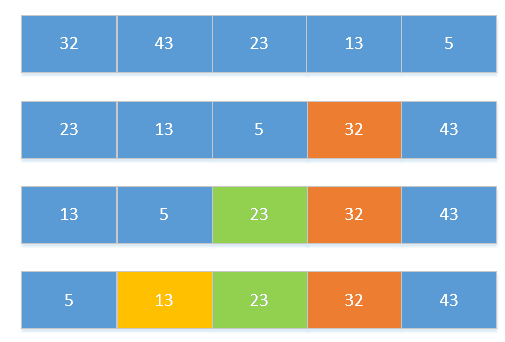``````public static void quickSort(int[] numbers, int start, int end) {
if (start  base) && (j > start))
j--;
if (i  i)
quickSort(numbers, i, end);
}
}  ``````

### 七、归并排序

1. 选择相邻两个数组成一个有序序列。

2. 选择相邻的两个有序序列组成一个有序序列。

3. 重复第二步，直到全部组成一个有序序列。``````public static void mergeSort(int[] numbers, int left, int right) {
int t = 1;
int size = right - left + 1;
while (t ``````

### 八、把基数排序

1. 将所有的数的个位数取出，按照个位数进行排序，构成一个序列。

2. 将新构成的所有的数的十位数取出，按照十位数进行排序，构成一个序列。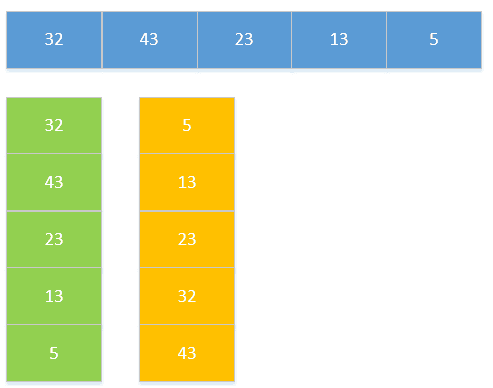``````public void sort(int[] array) {

int max = array;
for (int i = 1; i  max) {
max = array[i];
}
}
int time = 0;

while (max > 0) {
max /= 10;
time++;
}

List queue = new ArrayList();
for (int i = 0; i  queue1 = new ArrayList();
}

for (int i = 0; i  queue2 = queue.get(x);
queue.set(x, queue2);
}
int count = 0;

for (int k = 0; k  0) {
ArrayList queue3 = queue.get(k);
array[count] = queue3.get(0);
queue3.remove(0);
count++;
}
}
}
}``````